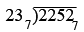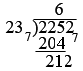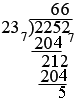SEARCH HOMEMath Central Quandaries & QueriesQuestion from MICHELLE, a student: DIVIDE 538 BY 14 IN BASE 2, 3, 4 & 5Michelle,

I'm going to illustrate the procedure by dividing 821 by 17 in base 7. I am going to first convert 821 and 17, both written in base 10, to base 7. This procedure is illustrated in my response to an earlier question. Here is my conversion of 821 to base 7

821 ÷ 7 = 117, remainder of 2
117 ÷ 7 = 16, remainder 5
16 ÷ 7 = 2, remainder 2
2 ÷ 7 = 0, remainder 2

Thus 821 = 22527. Similarly 17 = 237.

Before I actually start the division I am going to calculate 1× 237, 2 × 237, 3× 237 4× 237 5× 237 and

1 × 237 = 237
2 × 237 = 237 + 237 = 467
3 × 237 = 467 + 237 = 1027

and so on. My resulting table is

1 × 237 = 237
2 × 237 = 46 7
3 × 237 = 102 7
4 × 237 = 125 7
5 × 237 = 151 7
6 × 237 = 204 7

Now I can start the division.I can see from my multiplication that 237 divided 2257. 6 times. Thus I get, after subtractionAgain using of the table I see that 237 divided 2127 6 times and thusThus 22527 divided by 237 is 667 with a remainder of 57 .

PennyMath Central is supported by the University of Regina and The Pacific Institute for the Mathematical Sciences.